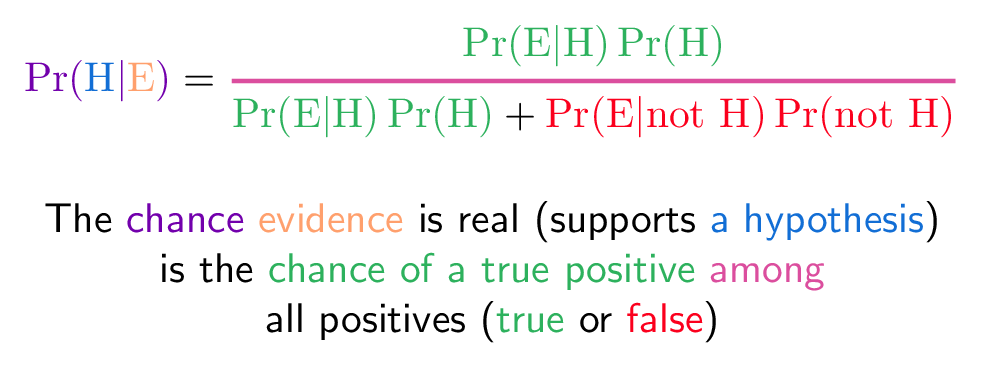# # Bayes' Theorem

## # Colorized Definition\newcommand{\chance}{\color{c1}}
\newcommand{\truepos}{\color{c2}}  % green (true)
\newcommand{\falsepos}{\color{c3}} % red (false)
\newcommand{\hypothesis}{\color{c4}}
\newcommand{\evidence}{\color{c5}}
\newcommand{\among}{\color{c6}}

$$\chance \Pr(\mathrm{ \hypothesis H}|\mathrm{ \evidence E}) \plain = \among \Frac{ \truepos \Pr(\mathrm{E}|\mathrm{H})\Pr(\mathrm{H}) }{ \truepos \Pr(\mathrm{E|H})\Pr(\mathrm{H}) \plain + \falsepos \Pr(\mathrm{E | not \ H})\Pr(\mathrm{not \ H})}$$

\plain      The
\chance     chance
\evidence   evidence
\plain      is real (supports
\hypothesis a hypothesis\plain) \\ is the
\truepos    chance of a true positive
\among      among \\
\plain      all positives (\truepos true \plain or \falsepos false\plain)